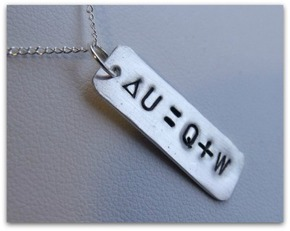# First Law of ThermodynamicsContent:

• Definition of The First Law of Thermodynamics
• Who Gave First Law of Thermodynamics?
• First Law of Thermodynamics Formula
• First Law of Thermodynamics: Example of Practical Use
• First Law of Thermodynamics, Video

## Definition of The First Law of Thermodynamics

The simplest definition of the first law of thermodynamics may sound like this: a change in the internal energy of a system is possible only under external influence. It is necessary to apply certain efforts from outside in order for some changes to occur in the system. These folk proverbs can serve as a peculiar expression of the first law of thermodynamics – “no water flows under a recumbent stone”, “you can’t easily pull a fish out of a pond” and so on. We can imagine that the fish is our closed system, any changes will not occur in it (the fish will not pull itself out of the pond) without our external influence and participation (efforts).

An interesting fact: the first law of thermodynamics explains why all many attempts to invent the “perpetual motion” had failed. Its existence is absolutely impossible according to the first law of thermodynamics

There was the simplest definition of the first law of thermodynamics at the beginning of our article. However, what does the first law of thermodynamics say besides this?

• Energy does not come from anywhere and does not disappear anywhere. It only transfers from one type to another (law of conservation of energy).
• The amount of heat received by the system goes to do its work against external forces and changes in internal energy.
• The change in the internal energy of the system during its transition from one state to another is equal to the sum of the work of external forces and the amount of heat transferred to the system and does not depend on the way this transition is carried out.
• The change in the internal energy of an open thermodynamic system is equal to the difference between the amount of heat transferred to the system and the work performed by the system on external forces.

## Who Gave First Law of Thermodynamics?

Around 1850 Rudolf Clausius and William Thomson (Kelvin) stated both the First Law – that total energy is conserved – and the Second Law of Thermodynamics.

## First Law of Thermodynamics Formula

What is the first law of thermodynamics equation? The formula or equation of the first law of thermodynamics can be written as follows:

Q = ΔU + W

The amount of heat (Q) transmitted to the system is equal to the sum of the changes in its internal energy (ΔU) and work (W).

## First Law of Thermodynamics: Example of Practical Use

The first law of thermodynamics has practical use to various processes in physics, for example, it allows us to calculate the ideal parameters of a gas in a variety of thermal and mechanical processes. In addition to practical use, this law can be applied in philosophy. Ecclesiastes (ancient scientist and philosopher) was the first man who wrote that nothing comes from nowhere or goes anywhere, but everything stays forever, constantly changing. This is the essence of the first law of thermodynamics.